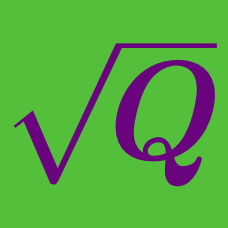Algebra

#### Challenge Quizzes

Solve the equation $\sqrt{ x+ 15 } = 9.$

Solve the equation

$\sqrt{ 6 x + 27 } = x.$

Solve for $x$:

$\sqrt{x+141} - \sqrt{ 5x+85} = 2$

The solution to the equation $1 + \sqrt{1 - \sqrt{x^4-x^2}} = x$ can be written in the form $\frac{a}{b}$ where $a$ and $b$ are coprime positive integers. Find $a+b$.

If $\frac{\sqrt{180+x}}{\sqrt{29}}=3,$ what is the value of $x$?

×